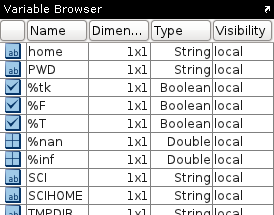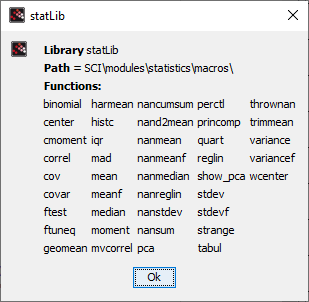# browsevar

Scilab variable browser

### Syntax

`browsevar()`

### Description

`browsevar` is the embedded Scilab variable browser.

`browsevar` lists all user variables the current environment.

`browsevar` has several features:

• A simple and comprehensive list• Easy tooltips on the dimensions or types• Sort by columns• Direct connection to the editvar component by double-clicking on the variable name

• Filter by type from the menu• Filter out Scilab predefined variables (SCI, SCIHOME, %pi, etc) from the menu### Examples

```a = rand(10,10);
b = "my string";
myCell = makecell([2 4],%t, int(-67),rand(2,3),1-%i,(1-%z)^3,1/(1-%z), "abcd", {"are", 45});
myList = list("Hello", grand(2,4,"uin",-100,100), [%t %f %f ]', (1-%z).^[1 2]);
myStru = struct("num",{3.1415, -1, %i}, "pol", {%z (1-%z) 2+%z^3}, "txt",{"Hi" "Hello" "Hola"});
function r=myTest(a, b), c = 2; r = a*c - b; endfunction
statLib = statisticslib;
plot2d()
curves = gce();

browsevar();```

Then click on some variables in the browser, to edit or display them.